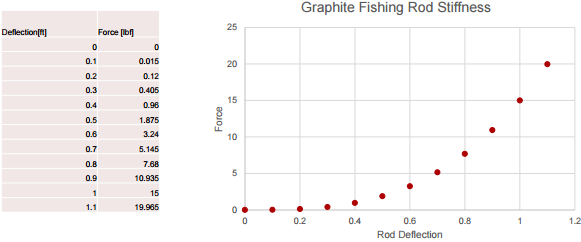+1-415-670-9189
info@expertsmind.com

# Get Solution

Calculate the cast distance of the rod
Course:- Physics
Reference No.:- EM131134399

 TweetExpertsmind Rated 4.9 / 5 based on 47215 reviews.
Review Site
Assignment Help >> Physics

Graphical Integration

1. Calculate the spring energy stored (in ft·lbf) in the rod just prior to release of the bait as a function of the bend in the rod (an expert caster will create maximum deflection in the rod).

2. Calculate the speed of the bait (in ft/s) assuming all the spring energy in the rod is transferred to the lure as Kinetic Energy. Assume the velocity imparted by the cast motion before the rod "snaps" is 44 ft/s, so the total energy of the lure is KE @ 44 ft/s + spring energy stored in rod.

3. Using a projectile calculator http://www.ajdesigner.com/phpprojectilemotion/range_equation.php

Calculate the cast distance of the rod.Minimize

Ask Question & Get Answers from Experts
Browse some more (Physics) Materials
 AN ELECTRIC MOTOR ACCELERATES THE 6-in DIAMETER GRINDING WHEEL AT A RATE 0F 150 rad/s2 FROM REST UNTIL IT REACHES ITS OPERATING SPEED OF 3600 rpm. DETERMINE: The time in sec You throw a ball from the top of a 50 m high building at 12 m/s upward. The ball misses the building on the way down. The mass of the ball is 2 kg. What is the total work do A capacitor is connected across the terminals of an ac generator that has a frenquency of 460 Hz and supplies a voltage of 24 V. When a second capacitor is connected in parall How many nitrogen molecules must strike a 1 cm2 surface each second to exert a pressure of 1 atm (Assume that the molecules are all moving at the same speed, at a temperature If the car is traveling a circle of 461 m, and the length (width) of the road is 14.6 m, what is the vertical height of road at the top of the bank in meters? Hint: find the Coherent light from a sodium-vapor lamp is passed through a filter that blocks everything except for light of a single wavelength. find the wavelength of the light that falls You are 1.9 m tall and stand 2.8 m from a plane mirror that extends vertically upward from the floor. On the floor 1.4 min front of the mirror is a small table 0.60 m high. By how much horizontal force would you need to apply to a 3.0 N plastic calculator to start it moving from rest? How much horizontal force would you need to keep it moving?